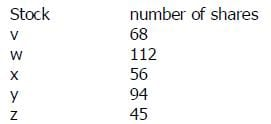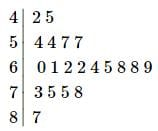# Test: Range

## 10 Questions MCQ Test Practice Questions for GMAT | Test: Range

Description
Attempt Test: Range | 10 questions in 10 minutes | Mock test for GMAT preparation | Free important questions MCQ to study Practice Questions for GMAT for GMAT Exam | Download free PDF with solutions
QUESTION: 1

Solution:
QUESTION: 2

Solution:
QUESTION: 3

### What is the range of a set consisting of the first 100 multiples of 7 that are greater than 70?

Solution:
QUESTION: 4

Set X consists of all two-digit primes and set Y consists of all positive odd multiples of 5 less than 100. If the two sets are combined into one, what will be the range of the new set?

Solution:

Set X = {11, 13, 17,............., 83, 89, 97}
Set Y = {5, 15, 25,..............., 75, 85, 95}

Combining two sets, say Set Z

Set Z = {5, 11, 13, 15, 17, 25,..................., 75, 83, 85, 89, 95, 97}

Range = Max Value - Min Value
Range (Z) = 97 - 5 = 92

QUESTION: 5

Set A consists of integers {3, -8, Y, 19, -6} and Set B consists of integers {K, -3, 0, 16, -5, 9}. Number Lrepresents the median of Set A, number M represents the mode of set B, and number Z = LM. If Y is an integer greater than 21, for what value of K will Z be a divisor of 26?

Solution:
QUESTION: 6

If a randomly selected non-negative single digit integer is added to set X {2, 3, 7, 8}, what is the probability that the median of the set will increase while its range will remain the same?

Solution:
QUESTION: 7The table shows the number of shares of each of the 5 stocks owned by Mr. Sami. If Mr Sami was to sell 20 shares of Stock X and buy 24 shares of stock y, what would be the increase in range of the number of shares of the 5 stocks owned by Mr Sami?

Solution:

Range before transaction:
112-45=67
Range after transaction:
(94+24)-(56-20)=118-36=82
The difference is: 82-67=15

QUESTION: 8

A set of 15 different integers have a range of 25 and a median of 25. What is greatest possible integer that could be in this set?

Solution:

Prior to median 25, there are 7 numbers.
To make the greatest number as greater as possible, these 7 numbers should cost the range as little as possible. They will be, 24, 23, 22, 21, 20, 19, 18.
So, the greatest value that can fulfill the range is: 18+25=43

QUESTION: 9

What is the range for the following data set:

1,2,8,9,7,4,1,1,3,2,3

Solution:

The range is the highest value number minus the lowest value number in a sorted data set:
1,2,8,9,7,4,1,1,3,2,3
We need to sort the data set:
1,1,1,2,2,3,3,4,7,8,9
range=9−1=8

QUESTION: 10

Below is the stem-and-leaf display of a set of test scores.What is the interquartile range of these test scores?

Solution:

The numbers in the "stem" of this display represent tens digits of the test scores, and the numbers in the "leaves" represent the units digits. This stem-and-leaf display represents twenty scores.

The interquartile range is the difference of the third and first quartiles.

The third quartile is the median of the upper half, or the upper ten scores. This is the arithmetic mean of the fifth- and sixth-highest scores. These scores are 73 and 69, so the mean is (73+69)÷2=71.

The first quartile is the median of the lower half, or the lower ten scores. This is the arithmetic mean of the fifth- and sixth-lowest scores. Both of these scores are the same, however - 57.

The interquartile range is therefore the difference of these numbers: 71−57=14Use Code STAYHOME200 and get INR 200 additional OFF Use Coupon Code